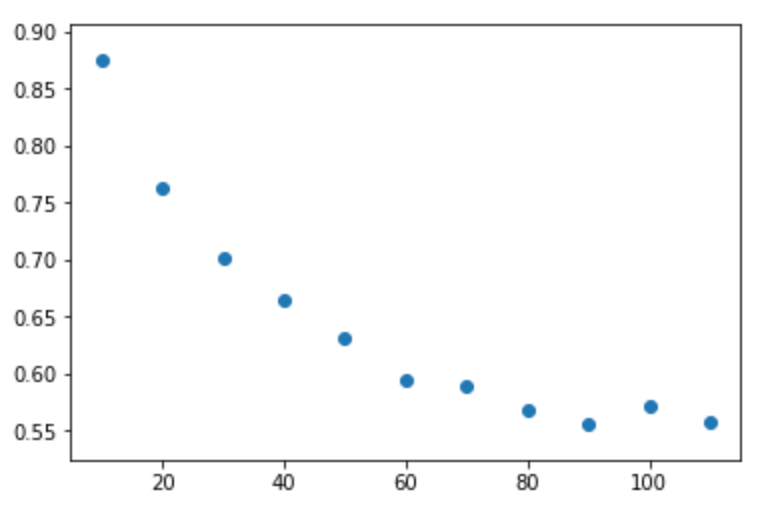# Basic Facebook Data Analysis with Python

The scandal with Cambridge Analytica, has produced no shortage of claims. Some have even gone as far as to blame the Trump victory and Brexit on it. Clearly, there’s more that will come to light. Only time will tell the full extent of the situation. However, I cannot help feeling that people are running to the most extreme conclusions in order to avoid responsibility or explain results that they didn’t see coming or understand. This doesn’t mean that fake news doesn’t plague Facebook, so let’s go through a small dataset of a couple of thousand political posts in the Trump and Clinton election posted by political groups that have been fact-checked: facebook-fact-check.csv

Because it’s a small dataset, we can be a bit rough and ready with the code. The speed of coding to get the basic stats, in this case, is worth the sub-optimal speed of running the code. In this example, we use the Pandas module as it’s very easy to filter and process data. First of all, we import the modules, define the main data frame, and calculate some basic percentages for mainly false and mainly true political posts (formatting is a bit strange, there is supposed to be an indent of four spaces under each colon in the code):

[code lang=text]
<br />Python Code

import pandas as pd
import pylab
import numpy as np
import matplotlib.pyplot as plt

len(main_data_frame[main_data_frame['Rating'] == 'mostly true']) / len(main_data_frame)

len(main_data_frame[main_data_frame['Rating'] == 'mostly false']) / len(main_data_frame)

[/code]

What this shows is that only 4% of the political posts are mainly false. Not really reflecting the hype and fear that the mainstream media is pushing. However, this doesn’t paint the full picture. Anyone who’s been on Facebook knows that all posts are not created equal. Some get barely noticed, whilst others are shared around and commented on, repeatedly hitting the top of your newsfeed. To look at this further, we can make two different data frames which are filtered. We then divide the sum of the shares by the number of posts in a data frame to get the basic averages:

[code lang=text]
<br />Python Code

mostly_true = main_data_frame[main_data_frame['Rating'] == 'mostly true']

mostly_false = main_data_frame[main_data_frame['Rating'] == 'mostly false']

mostly_false['share_count'].sum() / len(mostly_false['share_count'])

mostly_true['share_count'].sum() / len(mostly_true['share_count'])

[/code]

The average share count for a mostly true post is 1639, while the average share count for mostly false is 3535, that’s over double. With the same method above but changing the keys to “comment_count” we can see that the average comment count for false posts is 506, and 330 for true posts. So we’re not getting bombarded with fake posts, we just like to share and comment on them more.

Ok lets see if there’s any correlation between the number of shares and the probability of the post being correct. You can do this with a while loop. In the while, we increase the count by the bin size, create a data frame based on this, calculate the probability, and append this to a list. Realistically this is not optimum, but again considering how small the dataset is, it would take more time refining an elegant solution that smashing this out:

[code lang=text]
<br />Python Code

x_values = []
y_values = []

count = 0

while count <= 7000:
count += 50
split = main_data_frame[(main_data_frame['share_count'] < count) &
(main_data_frame['Rating'] != 'no factual content')]

split_two = main_data_frame[(main_data_frame['share_count'] < count) &
(main_data_frame['Rating'] == 'mostly true') ]
x_values.append(count)
y_values.append(len(split_two) / len(split))

[/code]

I played with the data and tried to put it into separate bins, however, the data points in the high end of shares becomes sparse so the data becomes noisy. As a result, the code generates a cumulative average. This artificially raises the probability, but smooths out the noise and gives the general trend giving the following result:

Here we can see the general trend being, the higher the number of shares, the lower the chance of the post being true. Similar trends can be observed with reaction count and comment count. With comment count there was enough for bins done by the following code:

[code lang=text]
<br />Python Code

x_values = 
y_values = 
counts = []

count = 0
index_count = 1

while count <= 100:
count += 10
split = main_data_frame[(main_data_frame['comment_count'] < count) & (main_data_frame['Rating'] != 'no factual content') & (main_data_frame['share_count'] >= x_values[index_count – 1])]

split_two = main_data_frame[(main_data_frame['comment_count'] < count) & (main_data_frame['Rating'] == 'mostly true') & (main_data_frame['share_count'] >= x_values[index_count – 1]) ]
x_values.append(count)
y_values.append(len(split_two) / len(split))
counts.append(len(split))
index_count += 1

del x_values
del y_values

[/code]

This resulted in the following binned (not cumulative):There were more comments but as the comment count increased, the number of posts in this category reduced to the point of noise setting in. As you can see, the more comments on a post, the lower the chance of the post being true.

Can we infer anything else? Not really. It could be that there’s an army of Russian bots trying to artificially raise the fake posts, it could just be that people feel more obliged to comment on a post that’s fake in order to set people straight, or it could be that the truth is uncomfortable so we avoid it. This data can’t explain the cause. We also have to remember that it’s a fairly small dataset, only two and a half thousand. But one thing is for sure, life is short and there is no shortage information on the internet. If the post has more than 20 posts on it, just scroll past.## maxwellflitton View All →

I help clinicians get to grips with coding and tech, I also code for a financial tech firm

This site uses Akismet to reduce spam. Learn how your comment data is processed.